# Multiplication and Division Fact Families

##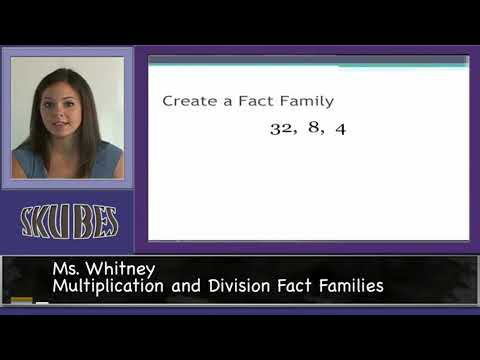By Skubes ed

3.OA.7 Fluently multiply and divide within 100, using strategies such as the relationship between multiplication and division (e.g., knowing that 8 × 5 = 40, one knows 40 ÷ 5 = 8) or properties# Multiplication and Division Relationships - Fun Math Videos for Kids 3rd Grade

##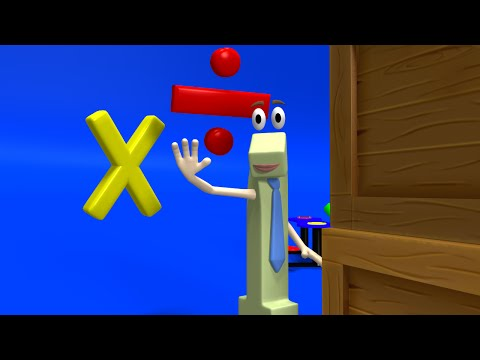By Math&learning videos

Multiplication and division relationships# Unknowns with multiplication and division | Multiplication and division | 3rd grade | Khan Academy

##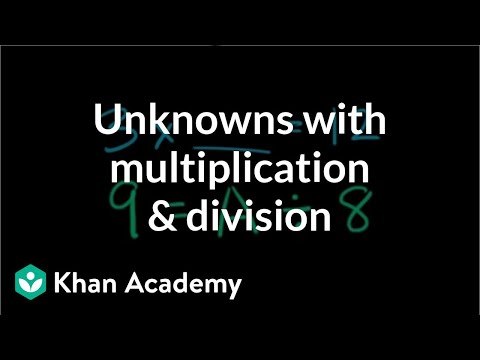By Khan Academy

Division of whole numbers# Connection between multiplication and division - 3rd grade math lesson

##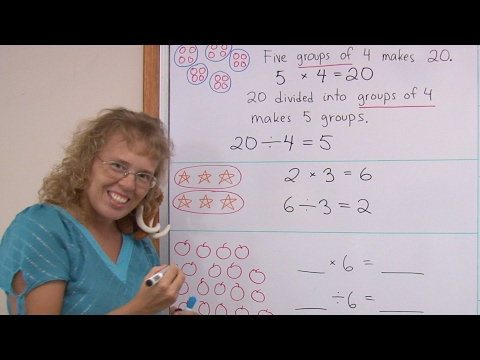By mathmamoth

For example, to solve 42 / 6, think how many times 6 makes 42 (in your six times table).# One-step equations with multiplication and division

##By Khan Academy

Remember that what you do to one side, you have to do to the other. Will you multiply or divide both sides to dump the fraction, x/a? Let's do it together.# One-step equations with multiplication and division

##By Khan Academy

This equation can be simplified through a single step to solve for the variable. Can you help?# One-step equations with multiplication and division

##By Khan Academy

Let's get a conceptual understanding of why one needs to divide both sides of an equation to solve for a variable.# One-step equations with multiplication and division

##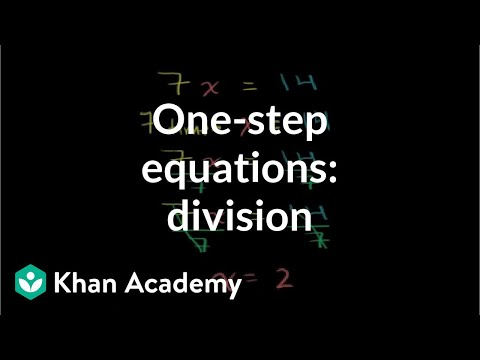By Khan Academy

Let's ease into this, shall we? Here's an introduction to basic algebraic equations of the form ax=b. Remember that you can check to see if you have the right answer by substituting it for the variable!# 2-digit times a 2-digit number | Multiplication and division | Arithmetic | Khan Academy

##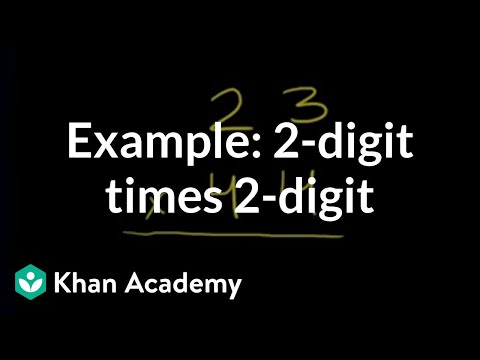By Khan Academy

In this interactive whiteboard demonstration the instructor shows how to multiply a five-digit number by a one-digit number. Produced by the Khan Academy. For fourth grades through sixth.# Grade 3 Math - Multiplication and Division Properties 1

##By Lumos Learning

Using the Lumos Study Programs, parents and educators can reinforce the classroom learning experience for children and help them succeed at school and on the standardized tests. Lumos books, dvd, eLearning and tutoring are used by leading schools, libraries and thousands of parents to supplement classroom learning and improve student achievement in the standardized tests.

PARENTS please visit LumosTestPrep.com to learn more.
EDUCATORS please visit LumosLearning.com to learn more# Division word problem example 1 | Multiplication and division | Arithmetic | Khan Academy

##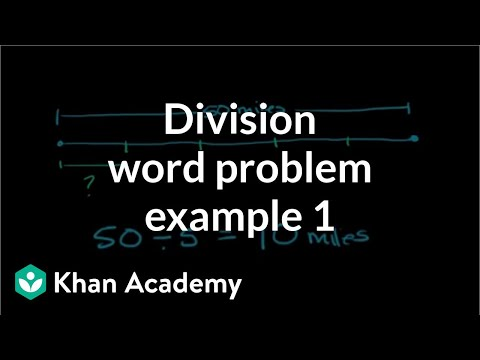By Khan Academy

Division of world problems# Multiplication word problem example 1 | Multiplication and division | Arithmetic | Khan Academy

##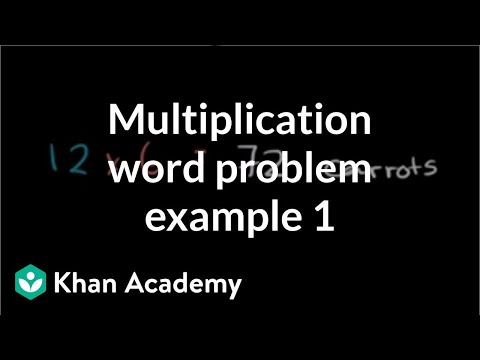By Khan Academy

Word problems on multiplication# Grade 3 Math - Multiplication and Division Facts

##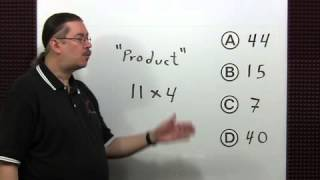By Lumos Learning

Using the Lumos Study Programs, parents and educators can reinforce the classroom learning experience for children and help them succeed at school and on the standardized tests. Lumos books, dvd, eLearning and tutoring are used by leading schools, libraries and thousands of parents to supplement classroom learning and improve student achievement in the standardized tests.

PARENTS please visit LumosTestPrep.com to learn more.
EDUCATORS please visit LumosLearning.com to learn more# One-step equations with multiplication and division

##By Khan Academy

This equation can be simplified through a single step to solve for the variable. Can you help?# One-step equations with multiplication and division

##By Khan Academy

Let's ease into this, shall we? Here's an introduction to basic algebraic equations of the form ax=b. Remember that you can check to see if you have the right answer by substituting it for the variable!# One-step equations with multiplication and division

##By Khan Academy

Let's get a conceptual understanding of why one needs to divide both sides of an equation to solve for a variable.# One-step equations with multiplication and division

##By Khan Academy

Remember that what you do to one side, you have to do to the other. Will you multiply or divide both sides to dump the fraction, x/a? Let's do it together.# Multiplication, division word problems: how many field goals? | Arithmetic | Khan Academy

##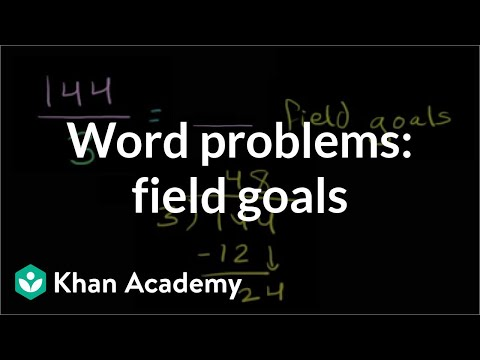By Khan Academy

It's time to start putting to practice the multiplication and division skills you've been building. Word problem fun!# One-step multiplication and division equations with fractions and decimals

##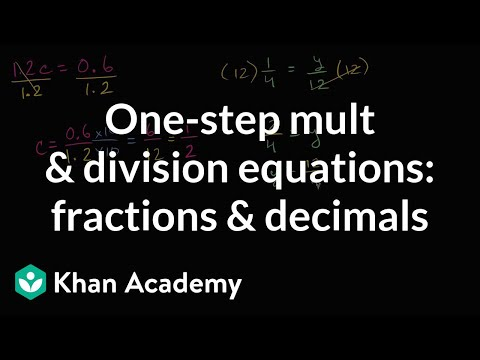By Khan Academy

Learn how to solve equations in one step by multiplying or dividing a number from both sides.ï¿½ï¿½ï¿½ï¿½ï¿½ï¿½ï¿½ï¿½ï¿½ï¿½ï¿½ï¿½These problems involve decimals and fractions.# Word Problems with Multiplication and Division

##By Mathademics

Word Problems with Multiplication and Division